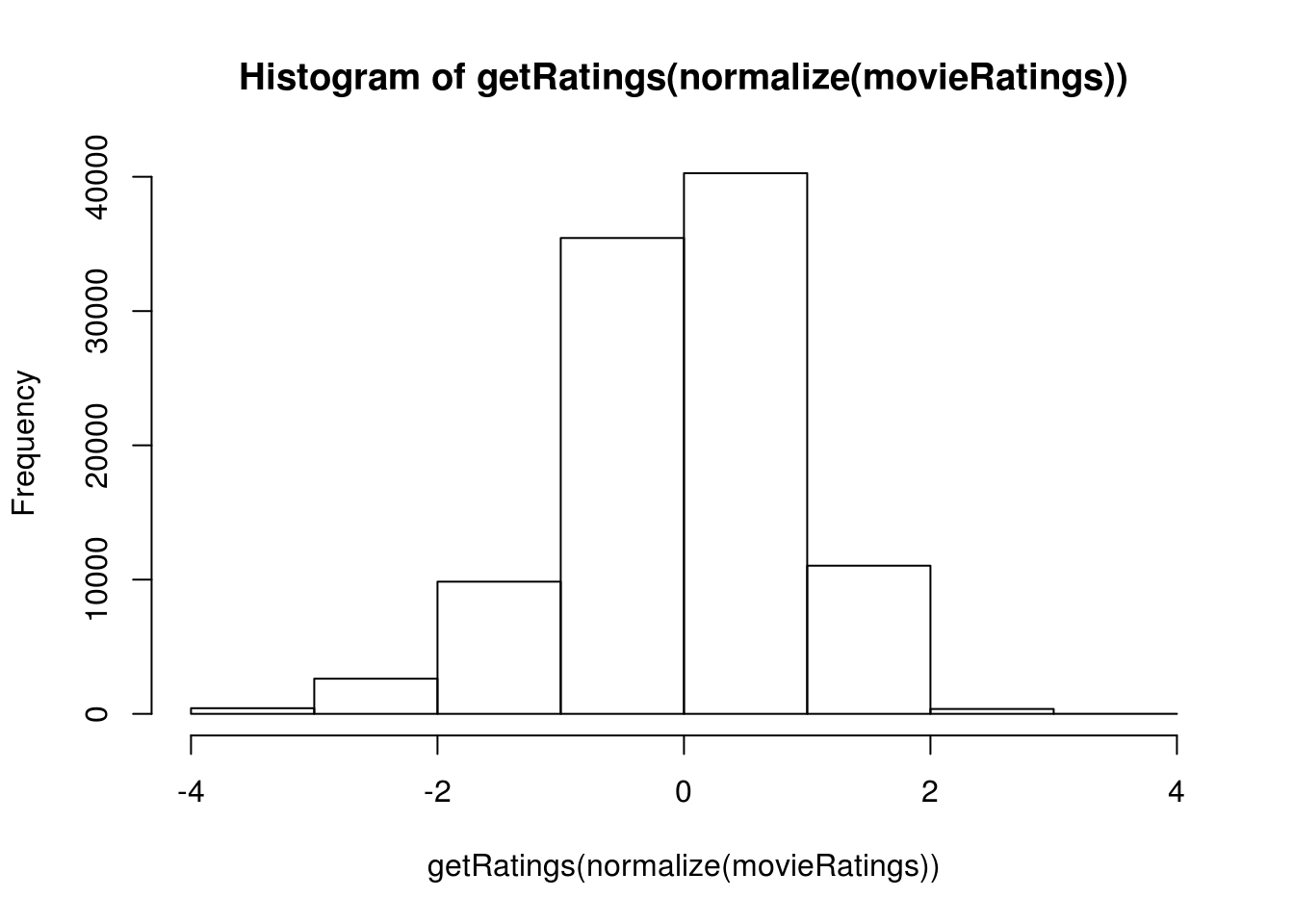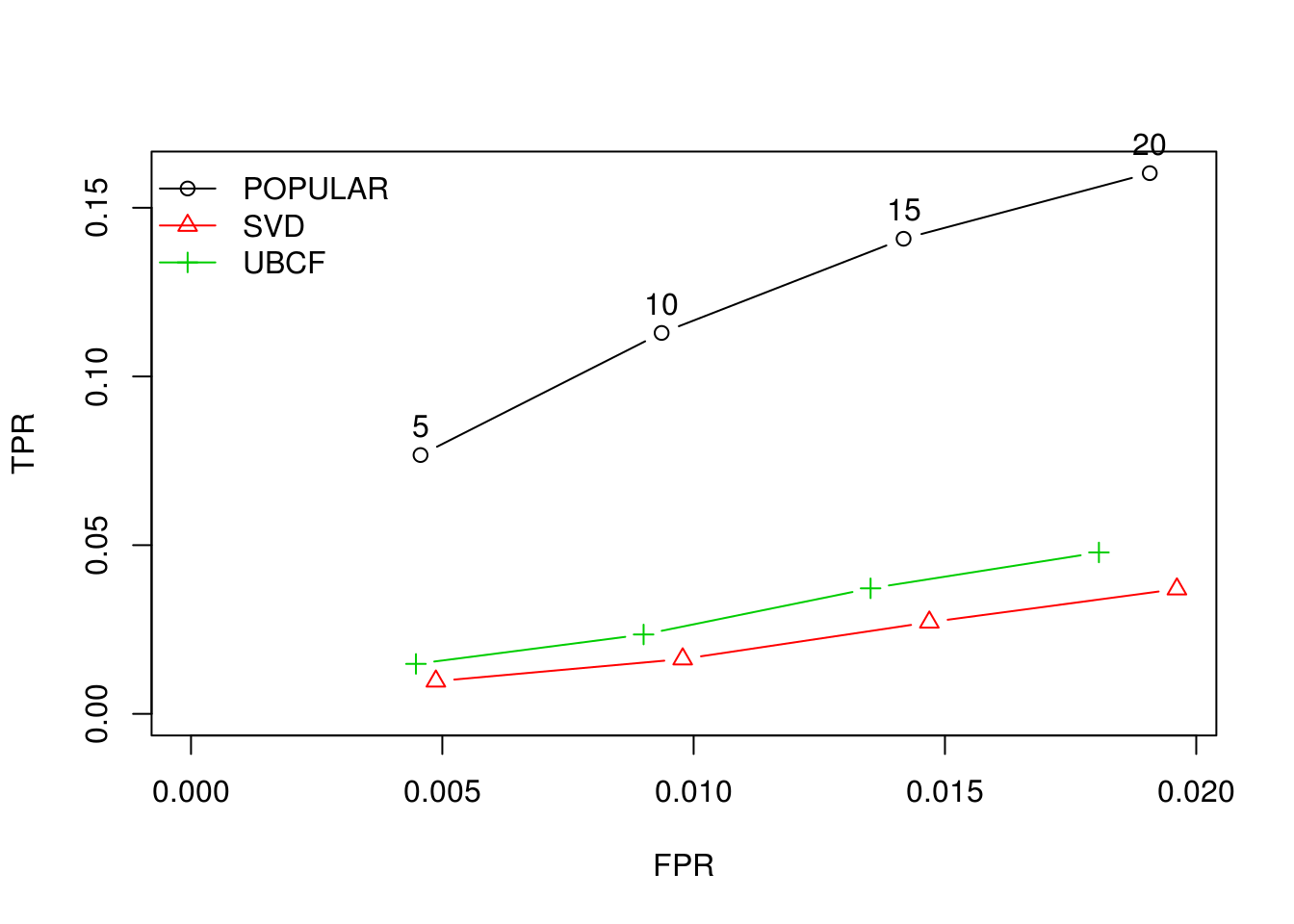Recommendation Engines in RIn this post, we will look at how to make a recommendation engine. We will use data that makes recommendations about movies. We will use the “recommenderlab” package to build several different engines. The data comes from

At this link, you need to download the “ml-latest.zip”. From there, we will use the “ratings” and “movies” files in this post. Ratings provide the ratings of the movies while movies provide the names of the movies. Before going further it is important to know that the “recommenderlab” has five different techniques for developing recommendation engines (IBCF, UBCF, POPULAR, RANDOM, & SVD). We will use all of them for comparative purposes Below is the code for getting started.

library(recommenderlab)

We now need to merge the two datasets so that they become one. This way the titles and ratings are in one place. We will then coerce our “movieRatings” dataframe into a “realRatingMatrix” in order to continue our analysis. Below is the code

movieRatings<-merge(ratings, movies, by='movieId') #merge two files
movieRatings<-as(movieRatings,"realRatingMatrix") #coerce to realRatingMatrix

We will now create two histograms of the ratings. The first is raw data and the second will be normalized data. The function “getRatings” is used in combination with the “hist” function to make the histogram. The normalized data includes the “normalize” function. Below is the code.

hist(getRatings(movieRatings),breaks =10)hist(getRatings(normalize(movieRatings)),breaks =10)We are now ready to create the evaluation scheme for our analysis. In this object we need to set the data name (movieRatings), the method we want to use (cross-validation), the amount of data we want to use for the training set (80%), how many ratings the algorithm is given during the test set (1) with the rest being used to compute the error. We also need to tell R what a good rating is (4 or higher) and the number of folds for the cross-validation (10). Below is the code for all of this.

set.seed(123)
eSetup<-evaluationScheme(movieRatings,method='cross-validation',train=.8,given=1,goodRating=4,k=10)

Below is the code for developing our models. To do this we need to use the “Recommender” function and the “getData” function to get the dataset. Remember we are using all six modeling techniques

ubcf<-Recommender(getData(eSetup,"train"),"UBCF")
ibcf<-Recommender(getData(eSetup,"train"),"IBCF")
svd<-Recommender(getData(eSetup,"train"),"svd")
popular<-Recommender(getData(eSetup,"train"),"POPULAR")
random<-Recommender(getData(eSetup,"train"),"RANDOM")

The models have been created. We can now make our predictions using the “predict” function in addition to the “getData” function. We also need to set the argument “type” to “ratings”. Below is the code.

ubcf_pred<-predict(ubcf,getData(eSetup,"known"),type="ratings")
ibcf_pred<-predict(ibcf,getData(eSetup,"known"),type="ratings")
svd_pred<-predict(svd,getData(eSetup,"known"),type="ratings")
pop_pred<-predict(popular,getData(eSetup,"known"),type="ratings")
rand_pred<-predict(random,getData(eSetup,"known"),type="ratings")

We can now look at the accuracy of the models. We will do this in two steps. First, we will look at the error rates. After completing this, we will do a more detailed analysis of the stronger models. Below is the code for the first step

ubcf_error<-calcPredictionAccuracy(ubcf_pred,getData(eSetup,"unknown")) #calculate error
ibcf_error<-calcPredictionAccuracy(ibcf_pred,getData(eSetup,"unknown"))
svd_error<-calcPredictionAccuracy(svd_pred,getData(eSetup,"unknown"))
pop_error<-calcPredictionAccuracy(pop_pred,getData(eSetup,"unknown"))
rand_error<-calcPredictionAccuracy(rand_pred,getData(eSetup,"unknown"))
error<-rbind(ubcf_error,ibcf_error,svd_error,pop_error,rand_error) #combine objects into one data frame
rownames(error)<-c("UBCF","IBCF","SVD","POP","RAND") #give names to rows
error
##          RMSE      MSE       MAE
## UBCF 1.278074 1.633473 0.9680428
## IBCF 1.484129 2.202640 1.1049733
## SVD  1.277550 1.632135 0.9679505
## POP  1.224838 1.500228 0.9255929
## RAND 1.455207 2.117628 1.1354987

The results indicate that the “RAND” and “IBCF” models are clearly worse than the remaining three. We will now move to the second step and take a closer look at the “UBCF”, “SVD”, and “POP” models. We will do this by making a list and using the “evaluate” function to get other model evaluation metrics. We will make a list called “algorithms” and store the three strongest models. Then we will make an objectcalled “evlist” in this object we will use the “evaluate” function as well as called the evaluation scheme “esetup”, the list (“algorithms”) as well as the number of movies to assess (5,10,15,20)

algorithms<-list(POPULAR=list(name="POPULAR"),SVD=list(name="SVD"),UBCF=list(name="UBCF"))
evlist<-evaluate(eSetup,algorithms,n=c(5,10,15,20))
avg(evlist)
## \$POPULAR
##           TP        FP       FN       TN  precision     recall        TPR
## 5  0.3010965  3.033333 4.917105 661.7485 0.09028443 0.07670381 0.07670381
## 10 0.4539474  6.214912 4.764254 658.5669 0.06806016 0.11289681 0.11289681
## 15 0.5953947  9.407895 4.622807 655.3739 0.05950450 0.14080354 0.14080354
## 20 0.6839912 12.653728 4.534211 652.1281 0.05127635 0.16024740 0.16024740
##            FPR
## 5  0.004566269
## 10 0.009363021
## 15 0.014177091
## 20 0.019075070
##
## \$SVD
##           TP        FP       FN       TN  precision     recall        TPR
## 5  0.1025219  3.231908 5.115680 661.5499 0.03077788 0.00968336 0.00968336
## 10 0.1808114  6.488048 5.037390 658.2938 0.02713505 0.01625454 0.01625454
## 15 0.2619518  9.741338 4.956250 655.0405 0.02620515 0.02716656 0.02716656
## 20 0.3313596 13.006360 4.886842 651.7754 0.02486232 0.03698768 0.03698768
##            FPR
## 5  0.004871678
## 10 0.009782266
## 15 0.014689510
## 20 0.019615377
##
## \$UBCF
##           TP        FP       FN       TN  precision     recall        TPR
## 5  0.1210526  2.968860 5.097149 661.8129 0.03916652 0.01481106 0.01481106
## 10 0.2075658  5.972259 5.010636 658.8095 0.03357173 0.02352752 0.02352752
## 15 0.3028509  8.966886 4.915351 655.8149 0.03266321 0.03720717 0.03720717
## 20 0.3813596 11.978289 4.836842 652.8035 0.03085246 0.04784538 0.04784538
##            FPR
## 5  0.004475151
## 10 0.009004466
## 15 0.013520481
## 20 0.018063361

Well, the numbers indicate that all the models are terrible. All metrics are scored rather poorly. True positives, false positives, false negatives, true negatives, precision, recall, true positive rate, and false positive rate are low for all models. Remember that these values are averages of the cross-validation. As such, for the “POPULAR” model when looking at the top five movies on average, the number of true positives was .3.

Even though the numbers are terrible the “POPULAR” model always performed the best. We can even view the ROC curve with the code below

plot(evlist,legend="topleft",annotate=T)We can now determine individual recommendations. We first need to build a model using the POPULAR algorithm. Below is the code.

Rec1<-Recommender(movieRatings,method="POPULAR")
Rec1
## Recommender of type 'POPULAR' for 'realRatingMatrix'
## learned using 9066 users.

We will now pull the top five recommendations for the first two raters and make a list. The numbers are the movie ids and not the actual titles

recommend<-predict(Rec1,movieRatings[1:5],n=5)
as(recommend,"list")
## \$`1`
##  "78"  "95"  "544" "102" "4"
##
## \$`2`
##  "242" "232" "294" "577" "95"
##
## \$`3`
##  "654" "242" "30"  "232" "287"
##
## \$`4`
##  "564" "654" "242" "30"  "232"
##
## \$`5`
##  "242" "30"  "232" "287" "577"

Below we can see the specific score for a specific movie. The names of the movies come from the original “ratings” dataset.

rating<-predict(Rec1,movieRatings[1:5],type='ratings')
rating
## 5 x 671 rating matrix of class 'realRatingMatrix' with 2873 ratings.
movieresult<-as(rating,'matrix')[1:5,1:3]
colnames(movieresult)<-c("Toy Story","Jumanji","Grumpier Old Men")
movieresult
##   Toy Story  Jumanji Grumpier Old Men
## 1  2.859941 3.822666         3.724566
## 2  2.389340 3.352066         3.253965
## 3  2.148488 3.111213         3.013113
## 4  1.372087 2.334812         2.236711
## 5  2.255328 3.218054         3.119953

This is what the model thinks the person would rate the movie. It is the difference between this number and the actual one that the error is calculated. In addition, if someone did not rate a movie you would see an NA in that spot

Conclusion

This was a lot of work. However, with additional work, you can have your own recommendation system based on data that was collected.